Divide number 135 into two additions so that one adds 30 more than 2/5 of the other add. Write the bigger one.

a =  75
b =  60

### Step-by-step explanation:

135 = a+b
b = 30 + 2/5•a

a+b = 135
2a-5b = -150

a = 75
b = 60

Our linear equations calculator calculates it.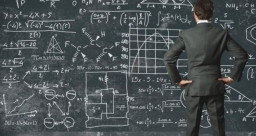Did you find an error or inaccuracy? Feel free to write us. Thank you!Tips to related online calculators
Need help to calculate sum, simplify or multiply fractions? Try our fraction calculator.
Do you have a system of equations and looking for calculator system of linear equations?

## Related math problems and questions:

• Reminder and quotientThere are given numbers A = 135, B = 315. Find the smallest natural number R greater than 1 so that the proportions R:A, R:B are with the remainder 1.
• Divisible by 5How many three-digit odd numbers divisible by 5, which are in place ten's number 3?
• Magic numberThe number 135 split to two addends so that one addend was 30 greater than 2/5 the addend.
• Ratio of two unknown numbersTwo numbers are given. Their sum is 30. We calculate one-sixth of a larger number and add to both numbers. So we get new numbers whose ratio is 5:7. Which two numbers were given?
• Three unknown numbers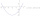The sum of of three numbers is 106. the first number is 10 more then the third. The second number is 2x the third. What are the numbers?
• RemaindersIt is given a set of numbers { 170; 244; 299; 333; 351; 391; 423; 644 }. Divide this numbers by number 66 and determine set of remainders. As result write sum of this remainders.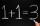Number 839 divide into the two addends that the first was 17 greater than 60% of the second. Determine these addends.
• Logistics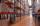Head of logistics department informed the meeting of management of the same item is currently not available in sufficient quantity in all three warehouses of the company. We have the total 135 pieces. It was 37 pieces more in the warehouse No. 3 than in t
• Resort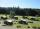In a completely crowded resort was 30% from the Czech Republic quarter visitors were from Slovakia and the rest 135 came from Germany. What capacity have resort?
• Sum of fall dices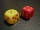What is the probability that the sum of 9 will fall on a roll of two dice? Hint: write down all the pairs that can occur as follows: 11 12 13 14 15. . 21 22 23 24. .. . 31 32. .. . . . . . .. . 66, count them, it's the variable n variable m: 36, 63,. .. .
• Two numbers 7The sum of two consecutive even numbers is 30. Find the numbers.
• Area 4gonCalculate the area of 4-gon, two, and the two sides are equal and parallel with lengths 11, 5, 11, and 5. Inner angles are 45°, 135°,45°, 135°.
• Unknown number 10Number first increased by 30%, then by 1/5. What percentage we've increased the original number?
• The sum 16The sum of A and B is 36. The difference of A & B is 8. find the larger number of A.
• PhotosThree friends Mirka, Lucka, and Hanka, collect photos. If Mirka had 3 more photos than she has, she would have as many as Hanka. If Lucka had 5 photos less than she has, she would be like Hanka. How many photographs does each have if they have 431 togethe
• The rawThe raw data presented here are the scores (out of 100 marks) of a market survey regarding the acceptability of new product launched by a company for random sample of 50 respondents: 40 45 41 45 45 30 30 8 48 25 26 9 23 24 26 29 8 40 41 42 39 35 18 25 35
• Evaluate 11Multiply the quotient of 6 and 2 by 3, then add 1. Add 1 and 6, then divide by 2 and multiply by 3. Divide 6 by the product of 2 and 3, then add 1.